Replication seems a sensible way to assess whether a scientific result is right. The intuition is clear: if a result is right, you should get a similar answer when repeating the work; if it it’s wrong, your answer should be quite different. Statisticians have devised numerous statistical tests for deciding whether a replication passes or fails thus validating of refuting the original result. I simulate many of these tests across a range of conditions. For exact replications, simple significance testing works fine as a validation test, but when replicas diverge from the original studies no test works well. Much of the replication literature focuses, perhaps unwittingly, on methods for testing whether the studies are similar; these tests work quite poorly under all conditions analyzed here.

## Introduction

The basic replication rationale goes something like this: (1) many published papers are wrong; (2) this is a serious problem the community must fix; and (3) systematic replication is an effective solution. (In recent months, I’ve seen an uptick in pre-registration as another solution. That’s a topic for another day.) In this post, I focus on the third point and ask: viewed as a statistical test, how well does replication work; how well does it tell the difference between valid and invalid results?

I consider a basic replication scheme in which each original study is repeated once.

Various authors have proposed tests for deciding whether a replication succeeds or fails. These include significance testing of the replica study, seeing whether the observed effect size of one study falls in the confidence or prediction interval of the other, checking whether the confidence or prediction intervals of the two studies overlap, significance testing of the studies combined via meta-analysis, and comparing the observed effect size of the replica study with a “small telescope” threshold derived from the smallest true effect size the original study could have plausibly detected.

I simulate these rules (and more) across many replication conditions and compute false positive and false negative rates across a range of conditions. The simulations vary the sample size from 10 to 1000 and true effect size from 0 to 1; in total, I simulate 14,641 conditions each with $$10^4$$ simulated replications, for a grand total of almost 150 million simulated instances.

A replication is exact if the two studies are sampling the same population and near-exact if the populations differ slightly. This is an obvious replication scenario. You have a study that you think may be a false positive; to check it out, you repeat the study, typically with a larger sample size, taking care to ensure that the replica closely matches the original.

A replication is inexact if the two studies are sampling very different populations. This scenario seems disconnected from the goal of validating an existing study. Since the populations are different, there’s no reason to expect the studies to get similar answers and little basis for declaring a study invalid if the replication fails. What motivates this scenario, I think, is generalizability not validity. You have a study that demonstrates an interesting effect in a limited setting and want to know whether it generalizes to other settings.

Significance testing of the replica works fine as a validation test for exact and near-exact replications, but error rates increase rapidly as the populations diverge. All other tests have excessive error rates under all conditions I analyzed. All tests have unacceptable error rates when used to check whether the original and replica studies are similar.

The software supporting this post is in my repwr GitHub repository. A document with technical details will soon be available in html and pdf on the GitHub Pages site associated with this repository.

## Literature

Here are the main papers and blog posts that led to this work.

## The Simulation

The software first simulates studies across a range of conditions, then combines pairs of studies into pairwise replications, applies rules (called measures) for deciding which pairwise replications pass, summarizes the results as counts and pass rates, and finally computes true and false positive and negative rates for measures and conditions of interest.

The studies are simple two group comparisons parameterized by sample size $$n$$ and population effect size $$d_{pop}$$ ($$d_{pop}\ge0$$). For each study, I generate two groups of random numbers, each of size $$n$$. One group, group0, comes from a standard normal distribution with $$mean=0$$; the other, group1, is standard normal with $$mean=d_{pop} \ge0$$. I then calculate basic statistics of interest, most notably the standardized observed effect size, $$d_{sdz}$$, aka Cohen’s d, as the mean of group1 minus the mean of group0 divided by the pooled standard deviation of the two groups. The software simulates many studies (default $$10^4$$) for each combination of $$n$$ and $$d_{pop}$$.

When I need to be pedantic, I use the terms study instance for each individual study and study set for the ensemble of study instances for a given combination of $$n$$ and $$d_{pop}$$.

The program varies $$n$$ from 10 to 1000 and $$d_{pop}$$ from 0 to 1. When analyzing results, I interpolate to get values that weren’t simulated directly.

To generate pairwise replications, I consider all (ordered) pairs of study sets. For each pair, the software permutes the instances of each study, then combines the instances row-by-row to get pairwise replication instances. It’s convenient to think of the first study of the pair as the original and the second as the replica.

A pairwise replication set is the ensemble of pairwise replication instances for a given pair of study sets. Four variables parameterize each pairwise replication set: $$n1$$, $$n2$$, $$d1_{pop}$$, $$d2_{pop}$$. These are, naturally enough, the sample and population effect sizes for the two study sets.

After forming the pairwise replications, I apply measures, i.e., rules for deciding which replications pass. Each measure takes a pairwise replication set as input and returns a vector of boolean values telling which instances pass or fail. The result is a boolean matrix whose rows represent instances and columns represent measures.

This post focuses on eight measures that seem most important.

• sig2 - the second study of the pair has a significant p-value
• sigm - the fixed effect meta-analysis of the studies has a significant p-value
• d1.c2 (resp. d2.c1) - $$d1_{sdz}$$ (resp. $$d2_{sdz}$$), the standardized observed effect size (aka Cohen’s d) of the first (resp. second) study is in the confidence interval of the second (resp. first) study; my implementation of confidence intervals is based on Uri Simonsohn’s code supporting this post
• d1.p2 (resp. d2.p1) - $$d1_{sdz}$$ (resp. $$d2_{sdz}$$) is in the prediction interval of the second (resp. first) study; my implementation of prediction intervals adapts code from David Stanley’s predictionInterval package
• c1.c2 (resp. p1.p2) - the confidence (resp. prediction) intervals of the two studies overlap

All measures assume that the first study is significant (sig1 in my notation) and the observed effect sizes of the two studies have the same sign (both positive or both negative).

I briefly examine Uri Simonsohn’s small telescopes method (d2.scp1 in my notation) from his paper and post. My implementation is based on Uri’s code supporting that post. Small telescopes, unlike the others, assumes that sig2 holds. For this reason, it needs a separate analysis.

The software summarizes the results by counting the number of positive results for each measure, taking into account the assumptions in the preceding paragraphs, and then converts the counts into pass rates. The final step is to convert pass rates into true positive, false positive, true negative, and false negative rates. This requires a definition of true and false instances, which in turn requires an explicit statement as to which replication instances represent replications that should succeed vs. ones that should fail.

## Correctness Criteria

I found no concise, rigorous definition of replication correctness anywhere. Many authors rely on some variant of “A replication should succeed if my method says so”. It’s impossible to define error rates with such circular definitions.

Replication researchers study two aspects of correctness.

1. The most basic concern is that the original study is a false positive.
2. The other concern is that the observed effect sizes of the two studies are inconsistent with each other. This might mean that the population effect sizes are different or that one result is an outlier.

The first concern seems sensible (at least, as sensible as any other use of the null hypothesis testing framework). The second concern baffles me: if the two studies are sampling different populations or one is an outlier, why does this invalidate the first study?

I’m tempted to ignore the second concern, but most proposed replication methods address it. Sadly, I’m stuck with it for purposes of this post.

Here are the precise technical definitions.

1. non-zero - a replication instance is true if $$d1_{pop}\ne0$$, i.e., the population effect size of the first study is non-zero
2. same-effect - a replication instance is true if $$d1_{pop}=d2_{pop}$$, i.e., both studies have the same population effect size; with tolerance $$\delta$$, a replication instance is true if $$abs(d1_{pop}-d2_{pop})\le\delta$$, i.e., the two population effect sizes differ by at most $$\delta$$

Note that these criteria depend only on the population effect sizes.

## Nomenclature

I’ve already introduced most of my nomenclature. Here is a concise reprise.

Studies

• study instance is a single simulated study
• $$n$$ is the sample size
• $$d_{pop}$$ is the population effect size
• $$d_{sdz}$$ is the standardized observed effect size (aka Cohen’s d)
• study set is the set of study instances for a given $$n$$ and $$d_{pop}$$

Pairwise replications

• pairwise replication instance (or simply replication instance) is an ordered pair of study instances
• s1 and s2 refer to the first and second study of the pair
• $$n1$$, $$d1_{pop}$$, $$d1_{sdz}$$ are the sample size, population effect size, and Cohen’s d of the first study
• $$n2$$, $$d2_{pop}$$, $$d2_{sdz}$$ are the sample size, population effect size, and Cohen’s d of the second study
• pairwise replication set (or simply replication set) is the set of replication instances for a given $$n1$$, $$n2$$, $$d1_{pop}$$, and $$d2_{pop}$$
• a replication instance or set is exact if $$d1_{pop}=d2_{pop}$$
• true and false refer to the answers given by correctness criteria when applied to replication instances: true instance is an instance for which the criterion returns true; false instance is one for which the criterion returns false
• positive and negative refer to the results of applying measures to replication instances: positive instance is an instance for which the measure returns true; negative instance is one for which the measure returns false
• true positive, false positive, true negative, and false negative combine the notions of true vs. false and positive vs. negative instance. For a given correctness criterion and measure
• true positive instance is a replication instance for which the correctness criterion and measure both return true
• false positive instance is an instance for which the correctness criterion is false, but the measure returns true
• true negative instance is an instance for which the correctness criterion and measure both return false
• false negative instance is an instance for which the correctness criterion is true, but the measure returns false

Correctness criteria

• non-zero is true if $$d1_{pop}=0$$
• same-effect is true if $$d1_{pop}=d2_{pop}$$; with tolerance $$\delta$$, same-effect is true if $$abs(d1_{pop}-d2_{pop})\le\delta$$
• for non-zero
• false positive occurs when $$d1_{pop}=0$$, but the measure returns true
• false negative occurs when $$d1_{pop}\neq0$$, but the measure returns false

-for same-effect with tolerance $$\delta$$ - false positive occurs when $$abs(d1_{pop}-d2_{pop})>\delta$$, but the measure returns true - false negative occurs when $$abs(d1_{pop}-d2_{pop})\le\delta$$, but the measure returns false

To simplify the presentation

• s1 (the first study of the replication instance) represents the original study and s2 (the second study) represents the replica
• error rates are relative to sig1; e.g., an error rate of 5% means 5% of the replications satisfying sig1 exhibit the error

## Graph Types

The parameter space is vast, because it’s possible to vary each of the four parameters ($$n1$$, $$n2$$, $$d1_{pop}$$, $$d2_{pop}$$) across a considerable range. I use several kinds of graphs to present the data in a comprehensive, yet concise, manner.

1. line graphs - simple and intuitive, I think, but not good at showing data that varies across many parameters
2. heatmaps - still reasonably intuitive and somewhat better at depicting multiple parameters
3. rate-vs-rate scatter plots - able to display error rates across large swaths of parameter space but with less parameter resolution and perhaps less intuitive clarity
4. aggregate line graphs - same data as rate-vs-rate scatter plots but for fewer measures and with better parameter resolution

## Results

### Replication used for validation

When replication is used to validate a study, non-zero is the correctness criterion that matters. I first present results for exact replications, then inexact, and finally near-exact.

#### Exact replications

Figures 1-4 show false positive rates for two values of $$n1$$ (20 and 40) and a range of values for $$n2$$. The smallest $$n2$$ in each case satisfies the recommendation in Uri Simonsohn’s post on the 90x75x50 heuristic that $$n2=2.5 \times n1$$ is big enough. The x-axis shows all four parameters using $$d1$$, $$d2$$ as shorthand for $$d1_{pop}$$, $$d2_{pop}$$ to conserve space. $$d1_{pop}=d2_{pop}=0$$ throughout because this is the only way to get false positives for non-zero with exact replications.

The first two figures are line graphs with false positive rate along the y-axis; these graphs use color and line width to distinguish the measures. The latter two are heatmaps with measures along the y-axis; these plots use color (shades of red and blue) to depict false positive rate; the switch from red to blue is set at the conventionally accepted threshold of 0.05 for false positives.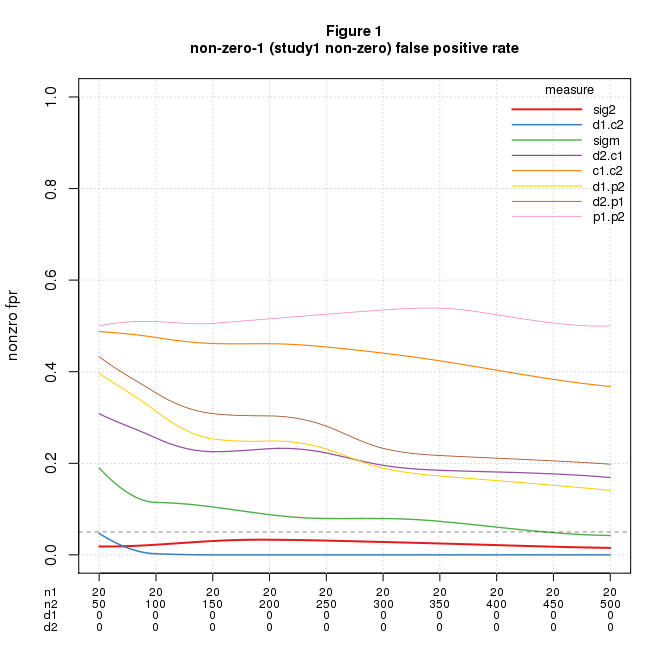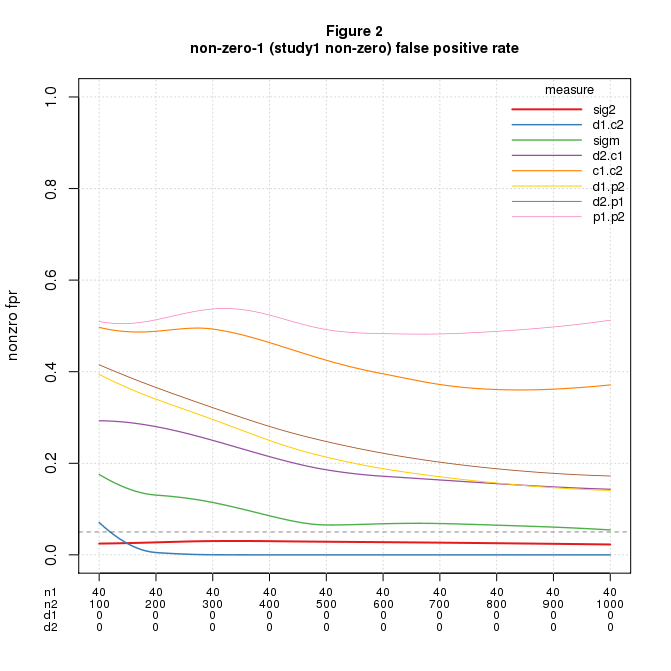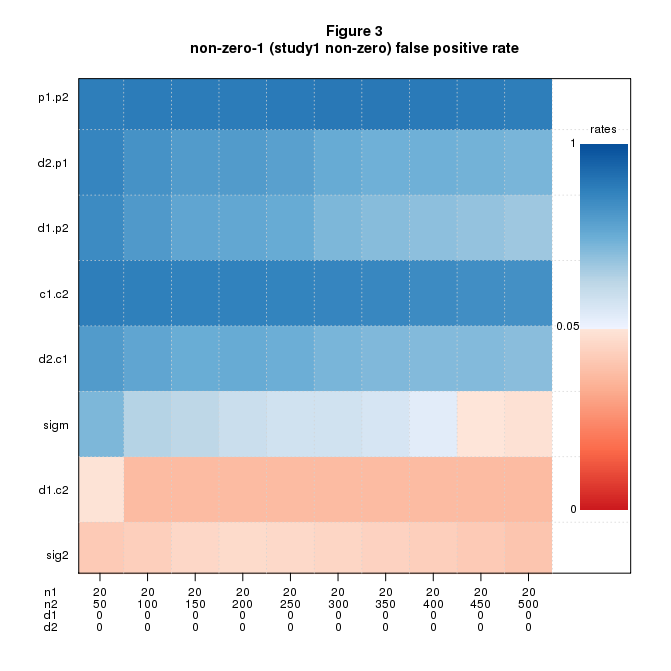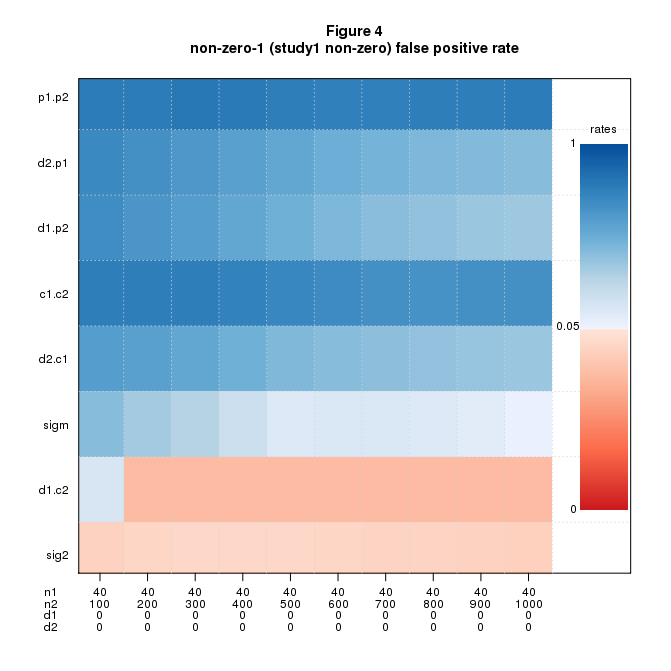The only measures with acceptable false positive rates across most of the parameter range are sig2 and d1.c2.

Plotting false negatives is more tiresome, because we can also vary $$d1_{pop}$$ (and simultaneously $$d2_{pop}$$, of course). Figures 5-6 are heatmaps of false negative rates for the same range of $$n1$$, $$n2$$ (but with a coarser grid of $$n2$$ values) and for $$d1_{pop}=d2_{pop}$$ varying over $$\{0.2, 0.5, 0.8\}$$. Each heatmap shows all three values of $$d_{pop}$$; the dark vertical lines visually split each plot into separate “panels” for each $$d_{pop}$$. The red-to-blue transition is set at the conventionally accepted threshold of 0.20 for false negatives.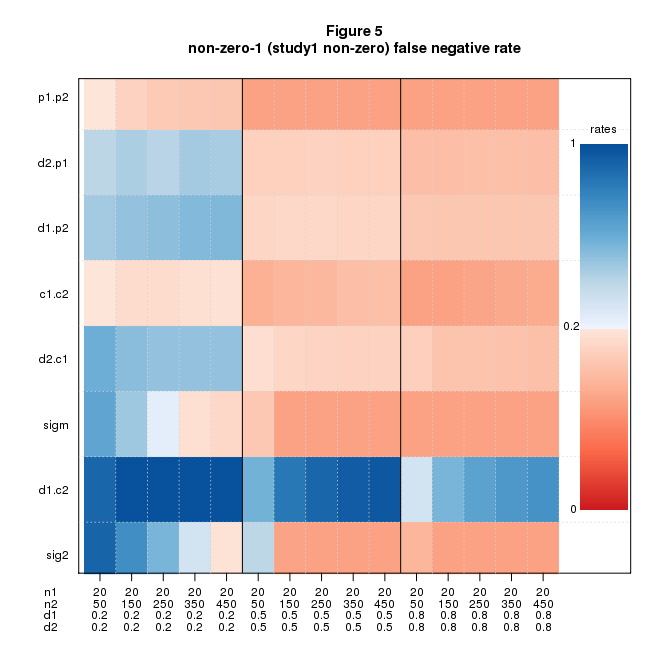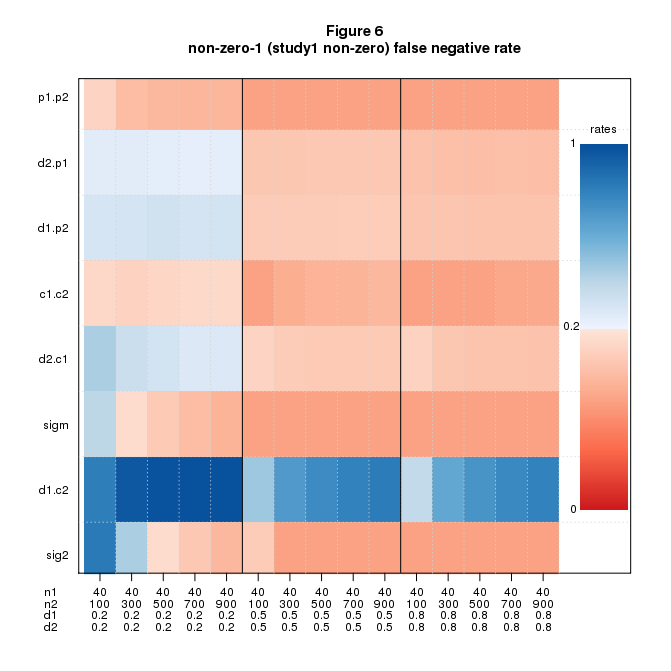As one would expect, performance improves as sample and effect sizes grow. The heatmaps for the larger sizes show a lot of red, telling us that many measures have acceptable false negative rates under these conditions.

For a measure to be usable, both error rates must be acceptable. Let’s compare the heatmaps for false positives and false negatives - figures 3-4 and 5-6. The only measure that is mostly red across all four figures is sig2; d1.c2, which looked good for false positives, is consistently bad for false negatives.

So far so good, but I’ve only shown data for a few values of $$n1$$. To see more conditions, I use a rate-vs-rate plot, inspired by receiver operating characteristic (ROC) curves, to plot false negative vs. false positive rates for a large range of conditions. See figure 7. The data in this figure extends the cases shown above: $$n1$$ varies from 20-160 in steps of 20, $$n2$$ is $$2.5\ or\ 5 \times n1$$, $$d1_{pop}$$ and $$d2_{pop}$$ range from 0 to 1 in steps of 0.1 with the constraint $$d1_{pop}=d2_{pop}$$. Each point shows the mean false negative vs. mean false positive rate for these conditions grouped by $$n1,n2$$. The rationale for grouping by $$n1,n2$$ is they are the only observable parameters. The dashed lines demark the conventionally acceptable error rates: 0.05 for false positives and 0.2 for false negatives; the bottom left hand corner is the region where both error rates are acceptable.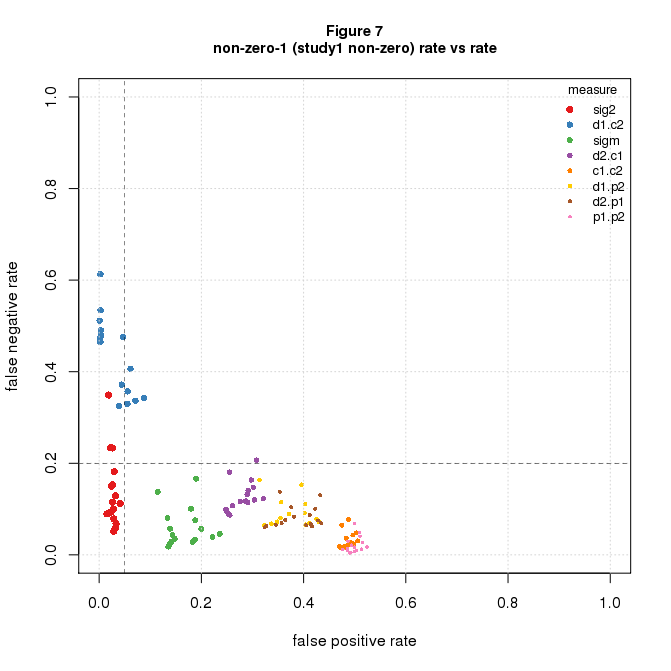The message clear: sig2 is the only measure with points falling in the acceptable range.

Boiling it down: for exact replications, sig2 is the only game in town. Its false positive rate is the significance level divided by 2 (the factor of 2 because it’s one-sided). The false negative rate is $$1-power$$ (the correlation of these statistics across the entire dataset is 0.99, data not shown).

#### Inexact replications

For inexact replications there are more cases to consider since $$d1_{pop}$$ and $$d2_{pop}$$ can vary independently. I’ll jump straight to rate-vs-rate plots to cover the ground succinctly using the same values of $$n1$$ and $$n2$$ as above, namely, $$n1$$ varies from 20-160 in steps of 20, and $$n2$$ is $$2.5\ or\ 5 \times n1$$.

Figures 8 shows the results: error rates are dreary in all conditions.